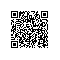# java中值得注意的

public class test {

public static void main(String[] args) {
int count=0;
int p=10;

for(int i=0;i<10;i++)
{

count=count++;

}
System.out.println(count);
}
}


分析：

p=count++;
count=p;


<pre name="code" class="java">如果是：
count=++count;

count++;使用钉钉扫一扫加入圈子
+ 订阅